# How to Calculate Weighted Average in ExcelClaudia Buckley#### Join the Excel conversation on Slack

Ask a question or join the conversation for all things Excel on our Slack channel.

I’m sure you’ve found the average of a group of numbers in the past. Some people can even do it subconsciously, giving you a rough estimate of the general tendency of the values in the distribution. Our resource on how to calculate an average in Excel covers seven different ways to do this.

However in some settings, we may consider some numbers within a group to be more significant than others, because of what they represent. This concept is known as a weighted average.

So how do you calculate weighted average in Excel? Well, there is no weighted average function per se, but Excel does have a way to work it out for you.

To explain how it works, let's take a look at some examples in our weighted average Excel guide. There are a variety of examples covered, so you'll be able to apply the principles to other situations you may encounter.

Use this free Excel file to practice along with the tutorial

## What is a weighted average?

In a typical situation, a teacher may give more weight to some tests or exams than others in order to come up with a final grade. You may get the following scores in a subject: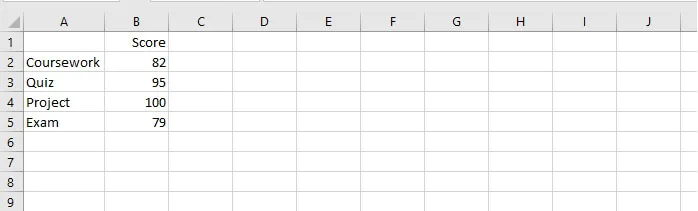To understand the concept of weighted averages, it’s important to remember that finding the regular average (arithmetic mean) of the above numbers is really saying that each number carries a value of a quarter (¼) of the whole. Adding all the numbers together and dividing by 4 gives each number equal weight, resulting in an average of 89.

So now let us introduce the idea of a weighted average.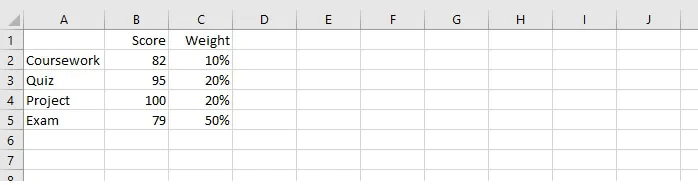The above weights tell us that even if the score on the coursework was 100, that would only value 10% of the final grade, and the score of 100 on the project will only be worth 20% of the final grade, instead of 25% in the previous example.

To arrive at the final score, we would multiply each score by its corresponding weight and then add the results. Excel has a function that does exactly that, and it’s called SUMPRODUCT.

SUMPRODUCT uses the values in at least two arrays or ranges, multiplies their values, and then returns the sum of their product. The SUMPRODUCT function can handle up to 256 arrays, and the syntax is as follows:

``=SUMPRODUCT(array1, [array2],...)``

Only one argument is required, but if you are using the SUMPRODUCT function, likely you have at least two. Arrays must be of the same size for the SUMPRODUCT function to work. Applying SUMPRODUCT to our example above will give us the following result.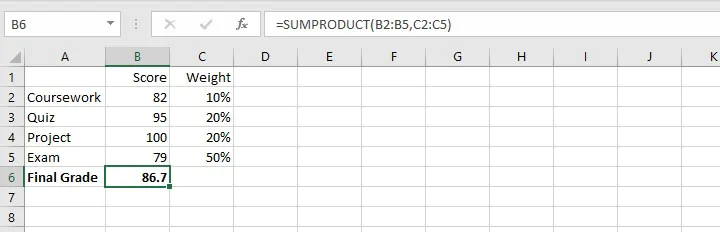## When weights do not add up to 100%

If we think of weights as priorities, we soon realize that listed weights do not always add up to 100%, because they may not, in fact, be listed as percentages.

For instance, companies may have various goals, each of which carry different overall priorities. Below is a sample of what this may look like.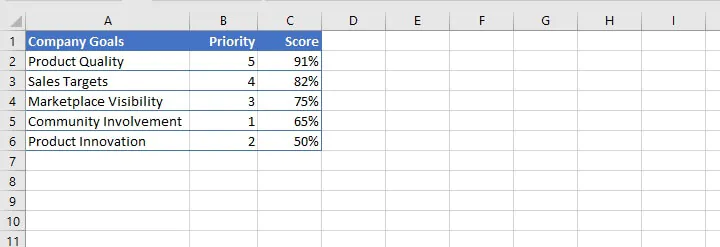Take note of two things in the priorities shown in column B:

1. They are expressed as whole numbers and do not add up to 100%.
2. The item considered highest priority is listed with the largest number, and the number with the lowest priority is assigned the smallest number.

It can be seen here that priority is just another word for weight. When we enter the score for each goal, there is a way to arrive at the company’s overall score for all goals as one figure.

However, if we simply applied the SUMPRODUCT function to the above ranges, we would get a result that really doesn’t mean anything because the priorities were not expressed as a fraction of a whole.

The simple solution is to divide that outcome by the sum of the priorities, or weights. This can be represented by the following syntax:

``=SUMPRODUCT(array1, [array2]...)/SUM(weights)``

If we apply this to our company goals scenario, we would enter:

``=SUMPRODUCT(B2:B6,C2:C6)/SUM(B2:B6)``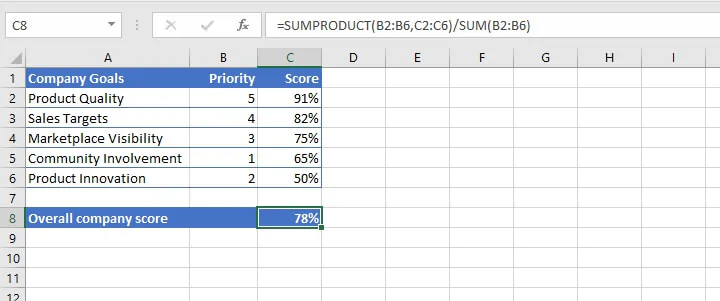The company can consider itself 78% on target for its goals.

## Weighted average inventory method

Weighted averages are also sometimes used in determining the value of inventory items purchased at different costs. Some companies prefer using the weighted average method over FIFO (first in, first out) or LIFO (last in, first out) because there may be no way to separate older items from newer ones.

The weighted average costing method involves working out an average cost per unit.

In the following example, identical spoons are purchased at different prices throughout the month but are all available for sale together.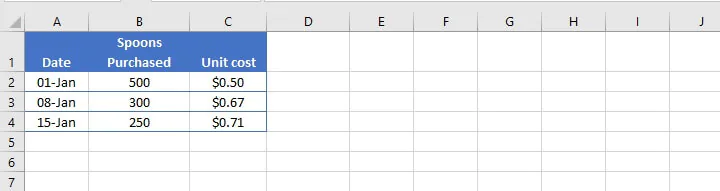It, therefore, makes sense to calculate the average cost since only one price will be set. The weighted average method works best in this situation.

The list of spoons purchased constitutes one array, and the list of unit costs is another array. Their SUMPRODUCT is divided by the sum of the number of spoons.

``=SUMPRODUCT(B2:B4,C2:C4)/SUM(B2:B4)``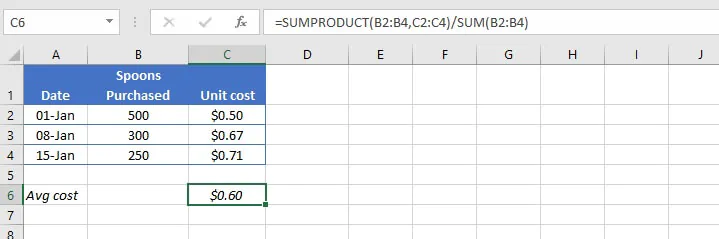Each spoon in the inventory costs \$0.60 and that can be used to determine the selling price.

## How to calculate weights

Here is a little trick for determining weights in strictly mathematical settings. A useful way to think about weights is with the idea of ratios. This is because weights do not always add up to a whole. This can be seen in the following example.

In an apartment building, the monthly maintenance fee of \$1000 is shared based on the number of occupants in each apartment. Each apartment’s weight is a fraction of the total number of occupants in the building.

Since a total of twelve people occupy the building, an apartment with one occupant carries a weightage of one-twelfth (⅟12) and would therefore pay one-twelfth of the maintenance fee. Apartments with two occupants would be two-twelfths, and so on.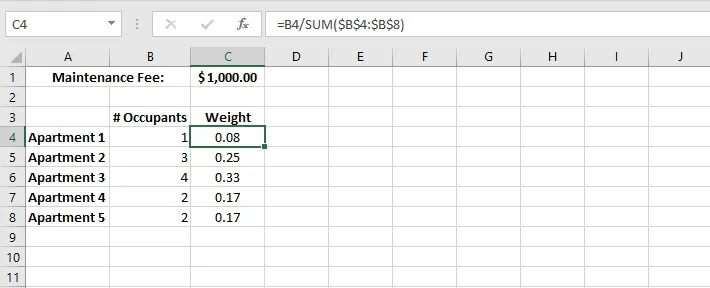In this case, the weights do add up to a whole, or 100%. Of course, calculating the amount due from each apartment would just be a matter of multiplying its weight by the total fee in cell C1 (\$1000).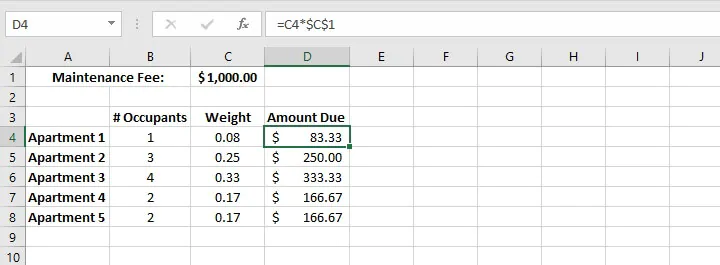That’s how you do a weighted average Excel style. Hungry for more?

Check out our Microsoft Excel - Basic and Advanced course to learn more useful formulas and functions that you can use to save time and boost your productivity in Excel. Or start with the free Excel in an Hour course for some basic formula knowledge.

Start learning formulas, functions, and time-saving hacks today with this free course!#### Join the Excel conversation on Slack

Ask a question or join the conversation for all things Excel on our Slack channel.Claudia Buckley

Claudia is a project manager and business skills instructor at GoSkills. In her spare time, she reads mystery novels and does genealogy research.